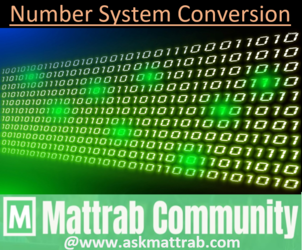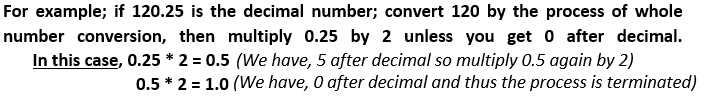# Decimal to Binary ConversionWhole Number Conversion
1. Divide the Decimal Number by 2 till the time it is divisible.
2. Note the remainders during successive division.
3. Write the remainders from the bottom(MSD) to the top(MSD).
The remainder listed in order as mentioned in step 3 is the equivalent Binary number of given Decimal Number.
Example:3. Continue the multiplication unless you get the part after decimal as 0.